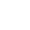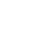# Parenthetic Doubt

### A newly discovered fragment of an early work by Descartes.

Translator’s Note. The following is translated from a manuscript found beneath the floorboards of a house in the Latin Quarter of Paris, used since 1615 as a brothel. On internal evidence it is ascribed to Descartes, and is believed to be a page from one of his early notebooks. It gives a fascinating insight into the mind and working method of a great philosopher, grappling with the problems that were later to receive their definitive Cartesian solutions in the Discourse on Method and the Meditations. — Andrew Belsey

I was sitting in my room late one night, meditating on what might possibly exist, and trying to write down my scattered thoughts as they occurred to my mind. Between my knees I was softening a lump of beeswax, and among the papers on the table in front of me was a hunk of coarse bread and an already half empty bottle of ruina matris, for the invention of which the enterprising Hollanders justly deserve praise. I had already to my own satisfaction, which is not to be taken lightly, and to the satisfaction of any man who possessed of the faculties of right reason is able to recognise the force of clear and distinct ideas, proved the existence of my own mind and of the Divine Mind (in that order), reasoning in a circle from the existence of a clear and distinct idea of God in my mind to the veritable existence of God himself, and from the existence of the Benevolent Deity to the veracity and trustworthiness of my clear and distinct ideas. ‘Tis very likely the ingenious and pious Father Mersenne and the subtle and atheistic Mr Hobbes will resist this reasoning, but to my mind and to God it is indubitable, and so I heed them not. But does my body exist? It seemed to my mind that indeed my body exists, and so I wrote down the proposition: “My body exists.” Undoubtedly my body exists. It therefore further seemed to my mind to be in accord with right reason to add the word “undoubtedly” to the afore-written proposition, thus: “My body undoubtedly exists.” But then a horrid doubt struck my mind. Was I unnecessarily laying myself open to the severe criticism of the ingenious and pious Father Mersenne and the subtle and atheistic Mr Hobbes? Surely they would say that what my proposition asserted as indubitable could be doubted. Then a solution came into my mind. Let the indubitability be asserted parenthetically, by placing parentheses around “undoubtedly”, thus: “My body (undoubtedly) exists.” But then another doubt struck my mind. Was I sure about the parenthetically asserted parentheses? But then another solution came into my mind. The parentheses themselves could be asserted parenthetically. This could be achieved by placing parentheses around the parentheses, thus: “(()” and “())”. Now my proposition was as follows: “My body (() undoubtedly ()) exists.” This seemed satisfactory, but then again a doubt struck my mind. Was I really content with the “(“s and the ”)”s around the “(“ and the ”)”? But then a solution came to my mind that roused me from my dogmatic slumber, if I may be permitted the use of a cant expression. The parenthetical mode is reiterable. Thus I could place parentheses around the parentheses around the parentheses. Now my proposition was as follows: “My body (()(()) undoubtedly (())()) exists.” This seemed satisfactory, and yet I feared that I was still not safe from the keen critical spirits of the ingenious and pious Father Mersenne and the subtle and atheistic Mr Hobbes. To make myself secure I conceived that it was necessary to reiterate the parenthetical assertion of the parenthetical assertion of the parentheses, and so to place parentheses around the parentheses around the parentheses around the parentheses. Now my proposition was as follows: “My body (()(())((())()) undoubtedly (()(()))(())()) exists.” This seemed satisfactory, and yet I felt still not securely on my ground. It was clearly demanded by right reason to reiterate the parenthetical assertion of the parenthetical assertion of the parenthetical assertion of the parentheses, and so to place parentheses around the parentheses around the parentheses around the parentheses around the parentheses. Now my proposition was as follows: “My body (()(()) ((())())((()(()))(())()) undoubtedly (()(())((())()))(()(()))(())()) exists.” Need I say that I found it absolutely essential to reiterate the parenthetical assertion of the parenthetical assertion of the parenthetical assertion of the parenthetical assertion of the parentheses, and so to place the parentheses around the parentheses around the parentheses around the parentheses around the parentheses around the parentheses. Now my proposition was as follows: “My body (()(())((())())((()(()))(())())((()(())((())()))(()(()) )(())()) undoubtedly (()(())((())())((()(()))(())()))(()( ())((())()))(()(()))(())()) exists.” And yet I still felt not secure with my proposition. Safety could be found, it seemed to my mind, only in reiterating the parenthetical assertion of the parenthetical assertion of the parenthetical assertion of the parenthetical assertion of the parenthetical assertion of the parentheses, and thus by placing parentheses around the parentheses around the parentheses around ….

[At this point the rest of the manuscript page is missing. When the text resumes on the verso it is clear that a certain amount of material is lost, but it does not appear to contain anything substantially different.]

… around the parentheses around the parentheses around the parentheses around the parentheses around the parentheses around the parentheses around the parentheses. Now my proposition was as follows: “My body (( )(( ))( (() )( ))( (() (( ))) (() )() )( (() (() )(( () )() ))( () (()))(())())((()(())((())())((()(()))(())()))(()(())((())()))(()(()))(()) ())((()(())((())())((()(()))(())())((()(())((())()))(()(()))(())()))(()(( ))((())())((()(()))(())()))(()(())((())()))(()(()))(())())((()(())((())() )((()(()))(())())((()(())((())()))(()(()))(())())((()(())((())())((()(()) )(())()))(()(())((())()))(()(()))(())()))(()(())((())())((()(()))(())())( (()(())((())()))(()(()))(())()))(()(())((())())((()(()))(())()))(()(())(( ())()))(()(()))(())()) undoubtedly (()(())((( ))())((()(()))(())())((()(())((())()))(()(()))(())())((()(())((())())((() (()))(())()))(()(())((())()))(()(()))(())())((()(())((())())((()(()))(()) ())((()(())((())()))(()(()))(())()))(()(())((())())((()(()))(())()))(()(( ))((())()))(()(()))(())()))(()(())((())())((()(()))(())())((()(())((())() ))(()(()))(())())((()(())((())())((()(()))(())()))(()(())((())()))(()(()) )(())()))(()(())((())())((()(()))(())())((()(())((())()))(()(()))(())())) (()(())((())())((()(()))(())()))(()(())((())()))(()(()))(())()) exists.” And yet I still felt not secure. Confound the ingenious and pious Father Mersenne and the subtle and atheistic Mr Hobbes! But at this point in my speculations my mind became aware that something sticky was running down my calves, and I discovered that the beeswax had melted. I scraped it up as best I could, spread it on the bread and consumed it, for my bodily appetite had become stimulated (by means as yet unknown to my mind) by this exercise of right reason. I washed it down with the remains of the ruina matris, and soon fell into a deep stupor. When I awoke I found it had all been a dream. Or had it? Yes, it had been a dream. Undoubtedly. It had undoubtedly been a dream. It had (undoubtedly) been a dream. It had (() undoubtedly ()) been a dream. It had (()(()) undoubtedly (())()) been a dream. It had (()(()) ….

[The rest is missing.]